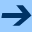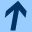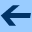Error-Correcting Linear Codes (Classification by Isometry and Applications)

## Error-Correcting Linear Codes (Classification by Isometry and Applications)

Springer, Berlin, Heidelberg, New York, 2006. ISBN: 3-540-28371-4.

Jointly written with ANTON BETTEN, MICHAEL BRAUN, ADALBERT KERBER, AXEL KOHNERT, and ALFRED WASSERMANN.This text offers a thorough introduction to the mathematical concepts behind the theory of error-correcting linear codes.

Care is taken to introduce the necessary algebraic concepts, for instance the theory of finite fields, the polynomial rings over such fields and the ubiquitous concept of group actions that allows the classification of codes by isometry. The book provides in-depth coverage of important topics like cyclic codes and the coding theory used in compact disc players.

The final four chapters cover advanced and algorithmic topics like the classification of linear codes by isometry, the enumeration of isometry classes, random generation of codes, the use of lattice basis reduction to compute minimum distances, the explicit construction of codes with given parameters, as well as the systematic evaluation of representatives of all isometry classes of codes. Up until now, these advanced topics have only been covered in research papers.

The attached compact disc contains numerical data, e.g. generator matrices of more than 70000 nonisometric optimal codes, as well as corresponding software which allows to evaluate generator matrices for given parameters, minimum distances, weight enumeration, the random generation of codes etc. The present book provides access to these results at a level which is suitable for graduate students of mathematics, computer science and engineering as well as for researchers.

For more information see: ERROR CORRECTING-LINEAR CODES: CLASSIFICATION BY ISOMETRY AND APPLICATIONS

harald.fripertinger "at" uni-graz.at, March 4, 2019